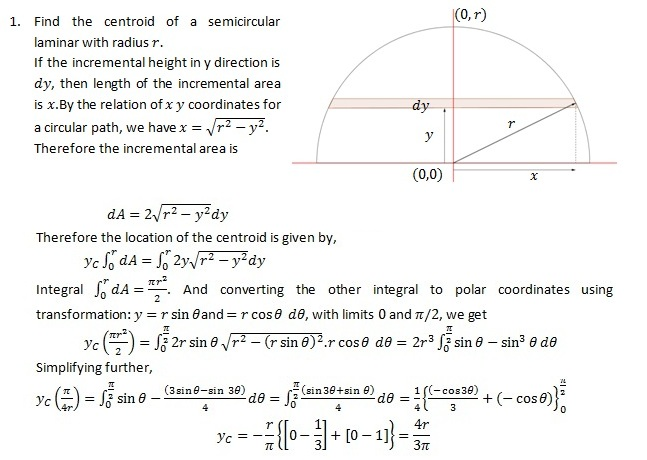# How to Find the Centroid

## What is Centroid

The centroid is the geometric center of a laminar object. It can also be described as the average position of all the points in a two-dimensional shape. For a laminar object with uniform density, when suspended through the centroid, object obtains equilibrium. The centroid of a convex object always lies within the perimeter of the object, while for a concave object the object may lies outside the perimeter. How to find the centroid of an object is explained below.

## Formulae to find the Centroid

The following formulae give coordinates of the centroid of an object.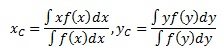Where f is the characteristic function of the geometric object,(A function that describes the shape of the object,product f(x) dx usually provides the incremental area of the object.

Therefore, it can be stated,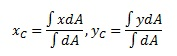If an object is a composition of several geometric objects, then it is easier to find the centroid of the composite object using the centroids of the individual components. If (xi,yi ) are the coordinates of the centroid of ith component and Ai is its area, then the centroid of the composite is given by,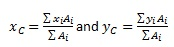If a composite includes an area removed, its area is taken as negative. Also, if the objects are symmetric, then the centroid lies on the axis of symmetry.

The position centroids of common geometric shapes are given below.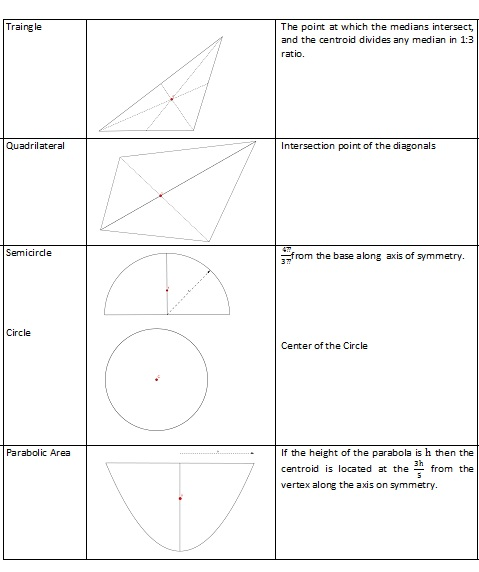Additionally, if the coordinates of the vertices of a triangle are(x1,y1),(x2 ,y2) and ( x3,y3) the coordinates of the centroid are given by xC=(x1+x2+x3)/3 and yC=(y1+y2+y3)/3

## How to find the centroid – Examples